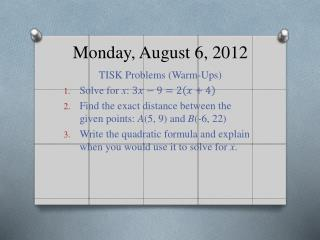DownloadDownload PresentationMonday, August 6, 2012

# Monday, August 6, 2012

Télécharger la présentation## Monday, August 6, 2012

- - - - - - - - - - - - - - - - - - - - - - - - - - - E N D - - - - - - - - - - - - - - - - - - - - - - - - - - -
##### Presentation Transcript

1. Monday, August 6, 2012 TISK Problems (Warm-Ups) Solve for x: Find the exact distance between the given points: A(5, 9) and B(-6, 22) Write the quadratic formula and explain when you would use it to solve for x.

2. Pass in Your Homework • Remember to pass homework in towards the center aisle. • Leave the homework in an organized stack on your table and I will pick it up.

3. Chapter 1: Discovering Points, Lines, Planes and Angles • This chapter will introduce you to Geometry. • You will learn some fundamental theorems which will be used throughout the rest of the year. • The concept you learn in this section will be deceptively easy, so make sure you are focused on truly understanding the idea and not just “how to get an answer”.

4. Lesson 1: Integration with Algebra In this lesson we’ll • Review how to graph ordered pairs on a coordinate plane And • Identify collinear points. • Discussion: Why do you think these are important ideas?

5. Let’s label some of the important features of the coordinate plane. First, what does it look like?

6. Discussion: What are ordered pairs and how do you use them?

7. What do you think the word “collinear” means?

8. Definition: Collinear • Collinear points are two (or more) points that lie on the same line. • Corollary definition: noncollinear points are two (or more) points that do NOT lie on the same line. • Any two points are always collinear since a line may be drawn between them.

9. How can you tell if three points are collinear… …given their coordinates? A(-1, 6) B(0, 3) C(2, -4) …given a graph?

10. Ways to Determine Collinear Points Given a Graph You must specify that collinear points have the same slope through any two points.

11. Ways to Determine Collinear Points Given Their Coordinates

12. Homework • p. 10 #26-36 even & 37 • You will need graph paper to complete this assignment. • If you don’t have graph paper, there is printable graph paper on my website under the Helpful Links section.